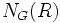# Weight for a finite group

## Definition

### Definition with symbols

let$p$ be a prime and$G$ a finite group. A weight of$G$ is a pair$(R,\phi)$ such that:

•$R$ is a$p$-subgroup of$G$, and in fact,$R = O_p(N_G(R))$ (viz it is the$p$-Sylow core of its normalizer$N_G(R)$).
•$\phi$ is an irreducible character of$N_G(R)$, the restriction of$\phi$ to$R$ is trivial, and$\phi$ belongs to a$p$-block of$N_G(R)/R$ of defect zero.

### Equivalence notion

Two weights are said to be equal if their is an inner automorphism of$G$ taking one to the other.

### Weights for a block

For$B$ a$p$-block of$G$, the weight is said to eb a$B$-weight if$B=b^G$ where$b$ is the associated block on$N_G(R)/R$.